# Experiment 7- Gravimetric Analysis of a Chloride Salt Flashcards

Set Details Share
created 8 years ago by brianquintero1
1,007 views
updated 8 years ago by brianquintero1
Page to share:
Embed this setcancel
COPY
code changes based on your size selection
Size:
X

1

Purpose of Experiment 7?

to illustrate typical techniques used in gravimetric analysis

by quantitatively determining

the amount of chloride in an unknown

2

What is quantitative analysis?

aspect of analytical chemistry concerned with determining

how much of one or more constituents is

present in a sample of material

3

Two common quantitative methods used in analytical chemistry

Gravimetric analysis

Volumetric analysis

4

Gravimetric analysis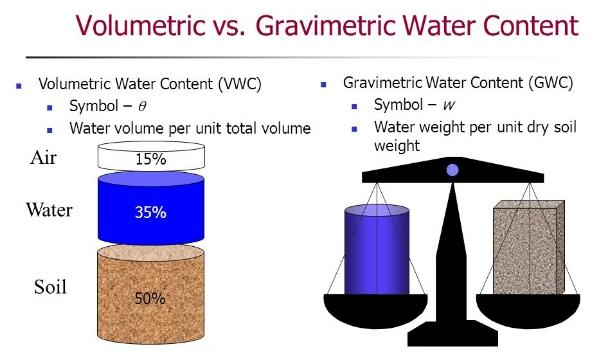derives name from the fact that

the constituent being determined can be in isolated

in some weighable form

5

Volumetric Analysisderives name from the fact that

the method used to determine amount of constituent involves

measuring the volume of a reagent

6

What are the steps involved in gravimetric analysis (7)?

(These are not steps, they are not are in order)

1. Dry and weigh samples of material

2. Dissolve the samples

3. Precipitate the constituent (substance you are trying to attain) by adding a suitable reagent

4. Isolate the precipitate by filtration

5. Wash precipitate to free it of contaminants

6. Dry the precipitate (to obtain weighable form)

7. Calculate the percentage of the desired consituent (from masses of the sample and precipitate)

7

Example of precipitation reactiona chloride ion may be precipitated by adding the silver ion to make AgCl

- AgCl is very insoluble, so adding Ag to Cl precipitates AgCl quantitatively

(the precipitate can be collected, dried, and weighed)

From the amount of AgCl obtained, amount of Cl can be calculated

8

Stoichiometrythe determination of the proportions in which elements combine

and the mass relations in any chemical reaction

9

gravimetric factor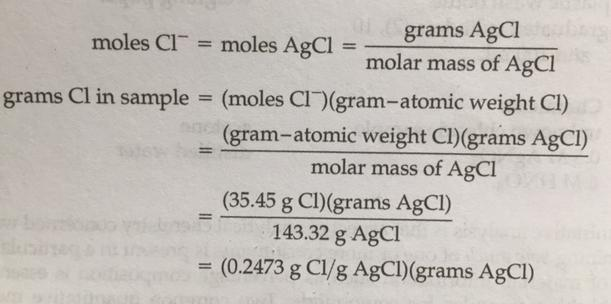- converts gram of a compound into grams of a single element

- used repeatedly in analytical chemistry and are tabulated in books

10

Example of Gravimetric Analysis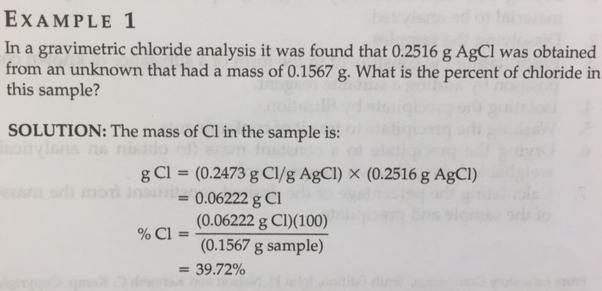11

Which acid was used in this procedure?

Which silver compound was used?

HNO3 - nitric acid

AgNO3- silver nitrate (.25M instead of .5 M)

12

How was the filter paper placed into the funnel?

open the paper so that it has one piece of paper against one side of the funnel and three pieces of paper against the other

- wet the paper w/ distilled water to hold it in place

13

Which compound was highly flammable and needed to be kept away from the flames?

acetone

14

What were the instructions for storing the AgCl precipitate? Why?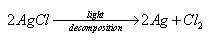Keep it out of bright light

because it is photosensitive and slowly decomposes in the presence of light

15

What is the purpose of standard deviation?

to estimate the precision of your results

The smaller the deviation, the more precise the measurements

16

accuracy

correctness of measurement,

closeness to true result

17

precision

internal consistency among one's own results (reproducibility)

18

error

difference btwn the true result and the determined result

19

Determinate errors

errors in method or performance that can be discovered and eliminated

*these errors are known and are controllable (e.g.instrumental errors, human errors)

20

Indeterminate errors

random errors, which are incapable of discovery but which can be treated by statistics

*these errors are unknown and beyond analysts' control (e.g. room temperature

21

mean

arithmetic mean or overage (μ)

22

median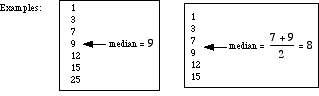midpoint of results (if odd number of results)

average of two middle results (if even number of results)

23

How is relative deviation calculated?

by dividing the average deviation from mean by the mean

24

What is the best measure of precision

standard deviation

25

What is the rule for retaining or discarding a standard deviation figure?

discard any result that is more than two standard deviations away from the mean